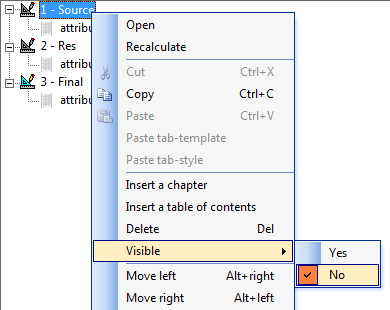Scroll

# Summing, Multiplying and Formatting Tables

Follow
 Summary This article is the second in a series of articles that will go through examples of using table arithmetic syntax new to Askia 5.3.5.0. This article will demonstrate the post processing or 'Cleaning script' and scripts in calculations (calculation arithmetic) which give the ability to freely create figures of your own, based on your current table or from a separate tab definition in your portfolio. Applies to Askia Analyse. Written for Researchers, Developers, Data Processors. Keywords Post processing, Cleaning script, Format, Layout, Colour, Conditional formatting, Table arithmetic, Forloops, Coordinates, data, Calculation arithmetic.

The .qes file and portfolio containing the examples discussed is attached: Table Arithmetic Examples 2.rar.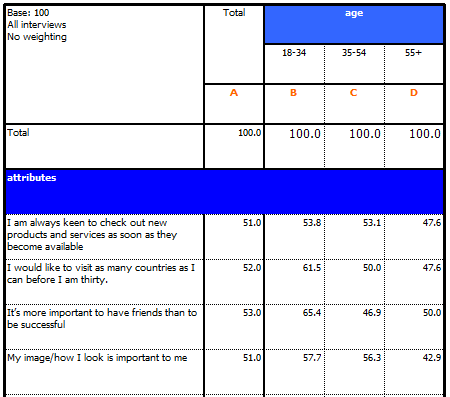The requirement is to create another table based off this which, for each cell, will perform a particular calculation:

((Sum of all scores in its column) * (Sum of all scores in its row)) / (Sum of all scores in table)

1. This is done in table (Res)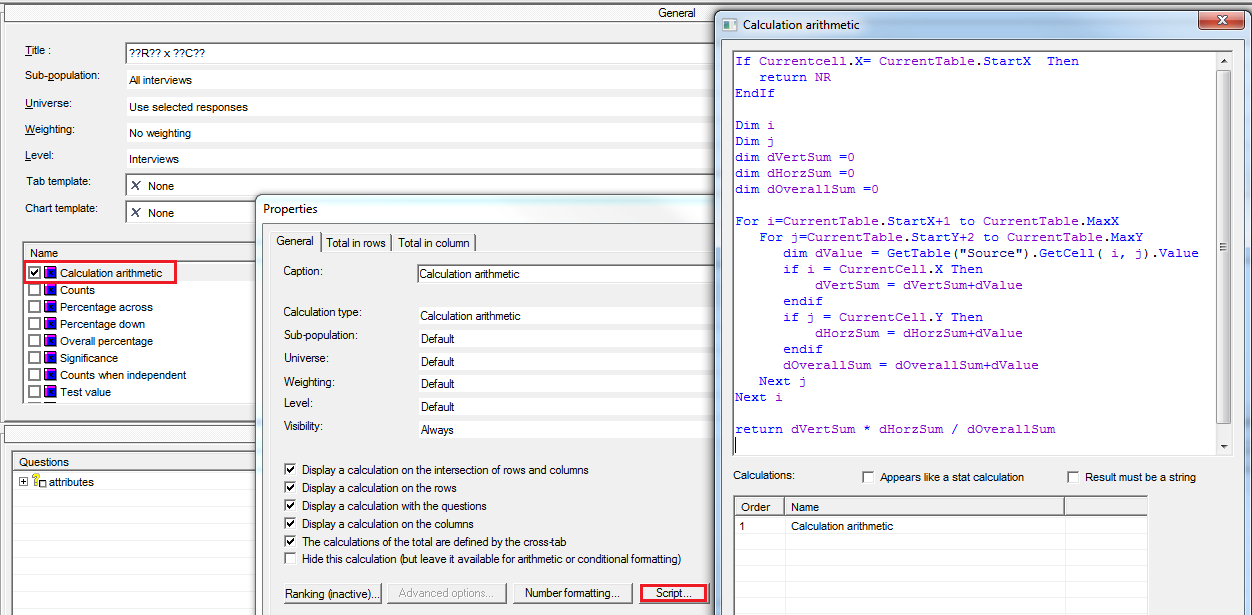1. Now we want to work out the deviation of the actual scores (Source) from the calculated norm scores (Res).

This is done in the table (Final)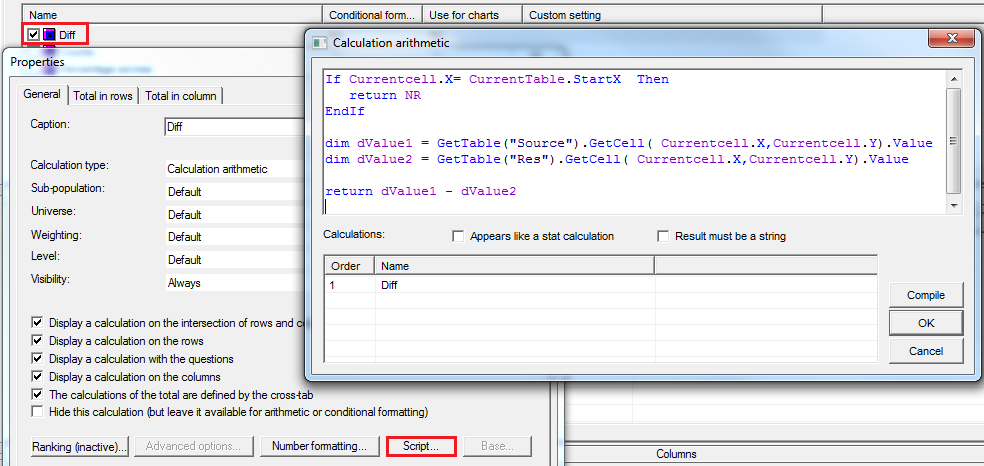1. The final requirement is to format the cell colour in the final table.
• If the difference is >=3 then colour amber
• If the difference is <=3 then colour sky blue
• Otherwise leave white

This is done by using the cleaning scripts, a kind of ‘post process’ that is run after the data is initially created in the table.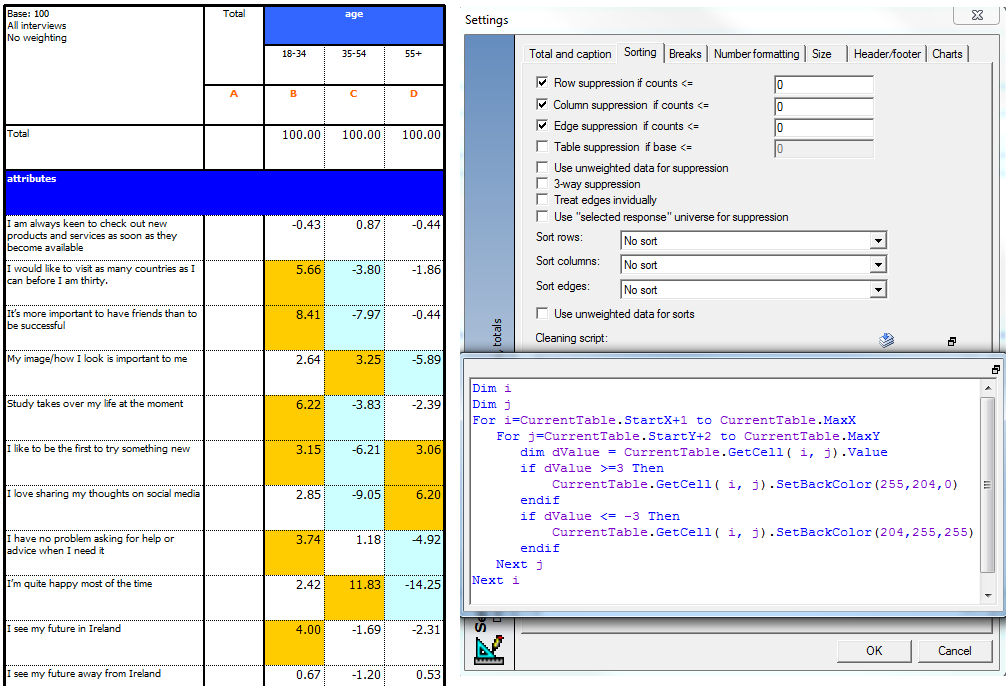In this particular portfolio we are only interested in the (Final) table. The tables before are only for getting to the end result and not to be seen in the final table set. So for this there is the option to make these tab definitions invisible: